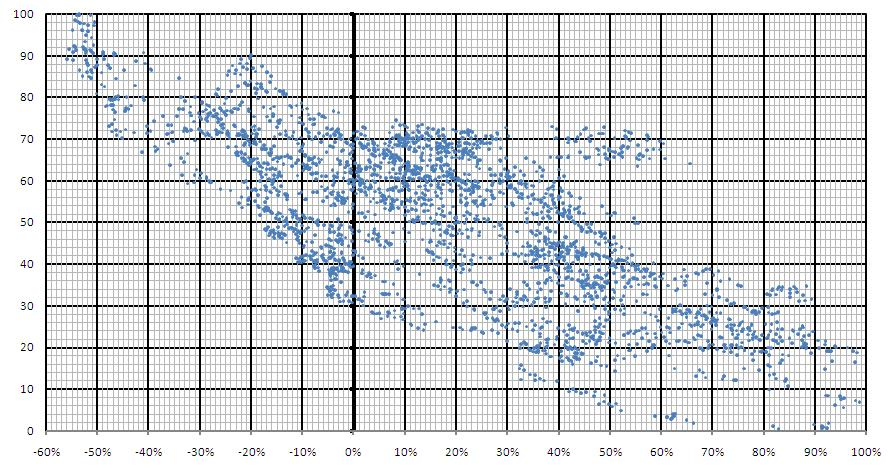Home >> FGI Data
RBI Repo Rate: Interest Rate at which the RBI lends money to banks. A key determinant of the cost of money in India.

RoE: Return on Equity. A measure of the capital efficiency of a business. Net Profit/ Net Worth expressed as a percentage. The number mentioned is the weighted average for Indian companies in the Nifty 50 Index.

PE Ratio
:Price to Earnings Ratio. Price Per Share/ Earning Per Share. The number mentioned is the weighted average for Indian companies in the Nifty 50 Index.

Dividend Yield: The dividend per share/ Price Per share expressed as a percentage. The number mentioned is the weighted average for Indian companies in the Nifty 50 Index.

_______________________________________________________________________-
 Author - FGI FrequencyPosted On 05 Aug 2011 This is a histogram which represents the frequency distribution of the FGI. The data represented is taken from 1st Jan 1999 till 1st July 2011. For example, here we see that for 14% of the data set the FGI is between 65 and 70. Author - FGI Line GraphPosted On 27 Jul 2011 In this comparative line graph the FGI is represented by the black line and the one year forward return of the nifty is represented by the red line. The mirror image created (although not perfectly so) implies a negative correlation between the two.  The higher the FGI the lower the returns and vice versa. Author - FGI Scatter GraphPosted On 27 Jul 2011 In this graph the FGI is plotted on the vertical axis and one year forward returns of the nifty are plotted on the horizontal axis. This set of data points are taken from 1st Jan 1999 till 1st July 2011. The pattern of dots slopes from the top left to the bottom right, thus suggesting a negative correlation. In other words, this graph shows that higher the FGI the lower the expected one year forward returns.